# How to Randomize a List in Google Sheets (3 Quickest Methods)

Google Sheets’s newly launched “Randomize Range” feature lets users randomize a list. You need to go to the main menu and click on “Data” followed by “Randomize range” to access this feature.

The Sort and Filter tools in Google Sheets are most commonly used to organize datasets in particular order.

However, there are a few scenarios where you may wish to sort the dataset in a completely random order. For example,

• Sorting student names in random order for group studies or games
• Picking up participants randomly for an event
• Selecting a winner for a giveaway

If you are searching for the quickest method to randomize a list in Google Sheets, then you have come to the right place.

In this article, we will discuss several methods to randomize a list in Google Sheets. It includes using built-in features and functions that may help you now as well as in the future, so make sure to read the article till the end.

Here are the three methods to randomize a list in Google Sheets. You can click on the links to jump to that particular section of the article.

• Using the Randomize range feature – (Quickest Method)
• Using the RAND function and Sort tool
• Using the ARRAYFORMULA function

### Copy the Example Google Sheet

Now, before we dive deep into each method of randomizing a list in Google Sheets, consider getting the following Google Sheets data and following me as I take you through this article.

The example Google Sheet contains datasets used to demonstrate various features and functions to randomize a list.

Let’s get started now without any further ado!

## How to Randomize a List in Google Sheets

As mentioned above, you can choose from various methods to randomize a list.

We will start with the quickest method to randomize a list, then dive deep into using the built-in functions to get almost similar results.

The good thing is you don’t need to be an expert to use any of these methods.

Here is the list of the Giveaway participants,

Task: Our job is to randomize the above list and pick up three winners.

Now, let’s get started.

### METHOD #1 – Using the Randomize range feature in Google Sheets to randomize a list

Google Sheets has recently announced this feature to let users quickly randomize datasets.

It is pretty straightforward. Before this announcement, users had to go through a comparatively lengthy workaround to randomize datasets.

Here are the steps,

• Select the range you wish to randomize
(Note that you need to avoid including the column header while selecting the range. In case you don’t have a header, then simply select the entire column and follow the steps below)
• Right-click to see more options
• Next, choose the “View more cell actions” option from the popup
• Click on “Randomize range

You will see that all the cells are shuffled randomly, as shown in the above GIF.

If you are not happy with the results, then make sure to use the “Randomize range” feature several times.

#### Q. What is another way to access the Randomize range feature in Google Sheets?

You have two options to apply the Randomize range to a list.

The first one is to right-click and select the “View more cell actions” option from the popup, as discussed above.

For the second one, here are the steps:

• Go to the main menu
• Click on the “Data” tab
• Select the “Randomize range” from the popup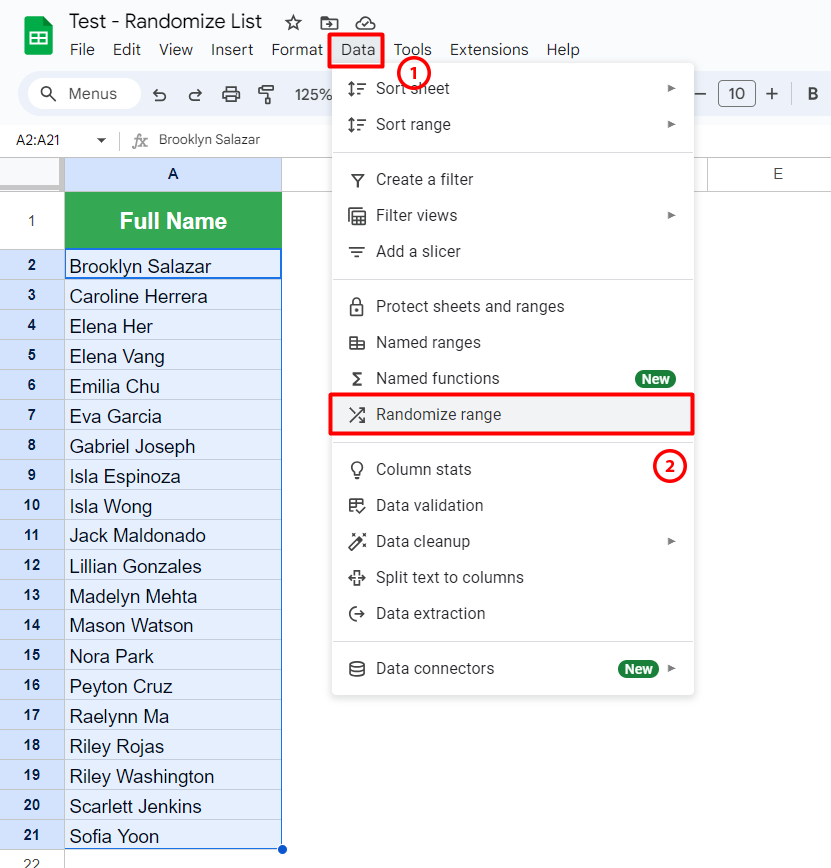Both of them require you to do a few clicks. So, you can choose it as per your preference.

### METHOD #2 – Using the RAND function and Sort tool in Google Sheets to randomize a list

This was the most popular method to randomize a list in the past. Before Google Sheets introduced the Randomize range feature, it was my go-to method.

In this method, you need to create a helper column and use the RAND function in Google Sheets to generate random numbers.

Those random numbers are then sorted in ascending or descending order using the Sort tool.

Before we dive deep into this method to randomize a list, let us have a quick look at the RAND function.

#### Explained: The RAND Function in Google Sheets

The RAND function generates a random number between 0 and 1 without any argument.

Here’s the general syntax for the RAND function,

``=RAND()``

This function automatically generates a new number each time you open the Google Sheet or perform any calculations within the spreadsheet. In other words, the calculations are real-time and vary depending upon the changes made to the Google Sheet.

Avoid inserting any values or providing the cell references for this formula, as it doesn’t accept them and returns an error.

That’s all about the RAND function.

Now, let us use it to randomize a list in Google Sheets.

#### Step 1: Prepare a helper column using the RAND function

We will start by adding a column next to the main list, as shown below.

You can name the column as per your preference. For the sake of simplicity, we have named it Helper.

Now, we will generate random numbers using the RAND function. Here are the steps:

• Click on the cell “B2
• Type “=rand
• Select the first option from the popup or press the “Tab” key
• Close the bracket using “)
• Press “Enter
• Hover to the lower-rightmost corner of cell B1 over the Fill Handle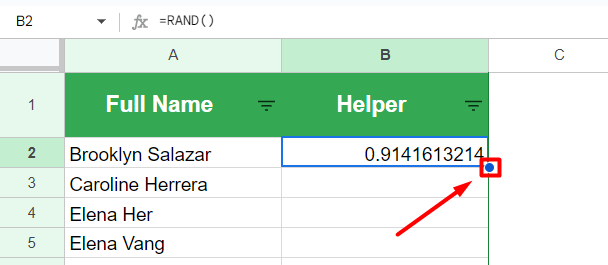• You will see a “+ icon
• Click on it and drag that “+” icon to the end of the table, as shown in the following GIF

Here’s how our helper column looks like,

Note that all the random numbers generated above would change each time you perform a certain calculation on them or even add new entries to the spreadsheet.

For example, I have added random input “asd” into the cell “D1“. As soon as I do it, the numbers are generated again. Refer to the following GIF.

#### Step 2: Randomize the dataset using the Sort tool

In this step, we will sort the entire dataset by the numbers generated using the RAND function.

Let’s begin,

• Select the entire table
• Hover to the toolbar section that sits right below the main menu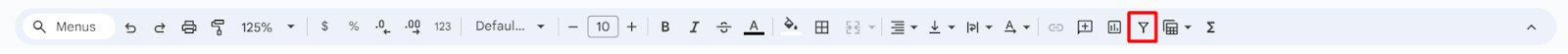• Click on the Filter icon
• Now, you will see a filter icon beside each column header, as shown below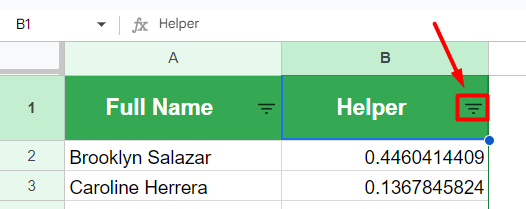• Go to the cell “B1” and click on the filter icon
• Select the first option, “Sort A to Z

Google Sheets will instantly sort the table based on current numbers generated using the RAND function. Also, at the same time, new numbers are generated, which can be used to randomize the list further.

The RAND is a volatile function that allows you to repeat the above process an infinite number of times to randomize a list.

### METHOD #3 – Using the ARRAYFORMULA function in Google Sheets to randomize a list

In this method, along with the ARRAYFORMULA, we need to use various built-in functions in Google Sheets.

We will combine all those functions to build a formula as follows,

``=SORT(A2:A21,ARRAYFORMULA(RANDBETWEEN(SIGN(ROW(A2:A21)),1000)),TRUE)``

It is a quite lengthy formula that randomizes a list every time you open the Google Sheets or perform any calculation within the spreadsheet.

Before we dive deep into the actual steps, let us quickly discuss each function used in the above formula so that you will understand how it is built.

#### Explained: The ARRAYFORMULA Function in Google Sheets

As from the name itself, it is an array formula that has the following general syntax.

``=ARRAYFORMULA(array_formula)``

The “arrray_formula” argument accepts cell range or an expression including the cell range as an input.

So, based on that particular cell range or array size, the function performs calculations. If you don’t put any expression (that includes the cell range), the function will simply display the entire cell range as is.

#### Explained: The SORT Function in Google Sheets

The SORT is a powerful function in Google Sheets. It allows users to sort datasets by single or multiple columns in ascending or descending order.

The general syntax for the SORT function is as follows,

``=SORT(range, sort_column, is_ascending)``

Here’s how to deal with each argument of the SORT function:

• range” – It needs to be replaced with the cell or table range, which needs to be sorted.
• sort_column” – Here, particularly in the case of the table, you need to define the column which you wish to sort.
• is_ascending” – This argument lets you decide if the sorting should be applied in ascending or descending order. It is an optional argument that accepts “TRUE” or “FALSE” as input.

Note that with the SORT function, you can sort the table using multiple columns.

#### Explained: The RANDBETWEEN Function in Google Sheets

This function is similar to the RAND function discussed in the second method of randomizing a list in this article.

The only difference is that the RANDBETWEEN function lets you generate random integer numbers within a specified range.

The general syntax for the RANDBETWEEN function is as follows,

``=RANDBETWEEN(low, high)``

The arguments are self-explanatory from the name itself.

• low” – Here, you need to define the lower bound of the range
• high” – It is the upper bound of the range

The function is pretty straightforward. Note that it is a volatile function that updates the random number each time you open the Google Sheet or perform any calculation within the spreadsheet.

#### Explained: The SIGN Function in Google Sheets

The SIGN function in Google Sheets is used to return the sign of a given number.

It evaluates the sign and returns “1” if it is positive or “-1” if it is negative. Whereas, for the zero as an input, the function returns “0”.

Here is the general syntax for the SIGN function in Google Sheets,

``=SIGN(value)``

Where the “value” argument needs to be replaced with the integer number whose sign needs to be evaluated. You can either manually enter the number or provide the cell reference holding the number.

It is combined with the other functions in Google Sheets to create powerful formulas.

#### Explained: The ROW Function in Google Sheets

The ROW is a simple lookup function in Google Sheets. It returns the location of a given cell or range of cells.

Simply put, the function returns the row number within a specific cell range.

The general syntax for the ROW function is as follows,

``=ROW([cell_reference])``

Here, the “cell_reference” argument is optional. If you don’t specify any value, then the function will return the row number where you have entered the formula.

You can provide a cell reference or range to this function.

Now that we have learned all the functions let’s combine them to create a formula capable of randomizing a list in Google Sheets.

• Click on the cell “B2
• Type “=sort
• Select the first option from the popup or press “Tab” key
• Provide the cell reference “A2:A21” for the “range” argument of the SORT function
• Press “,” to move to the next argument
• Type “arrayformula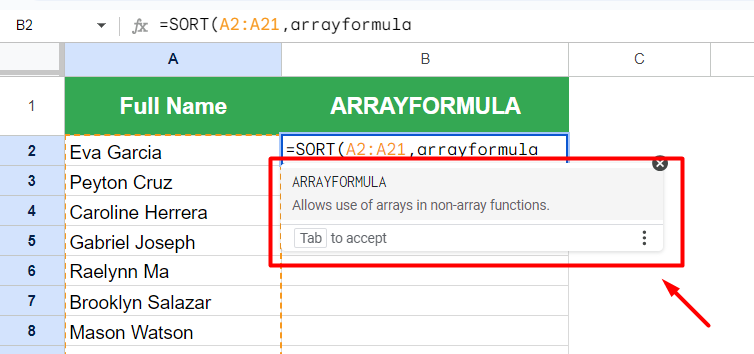(As the ARRAYFORMULA is a function in Google Sheets, you will see a popup like the above)
• Select the first option from the popup
• Now, for the “array_formula” argument of the ARRAYFORMULA function, we will type “randbetween
(Here, we are creating an expression using the RANDBETWEEN function. It will generate a random number for the cell range defined in the ARRAYFORMULA function)
• Select the first option from the popup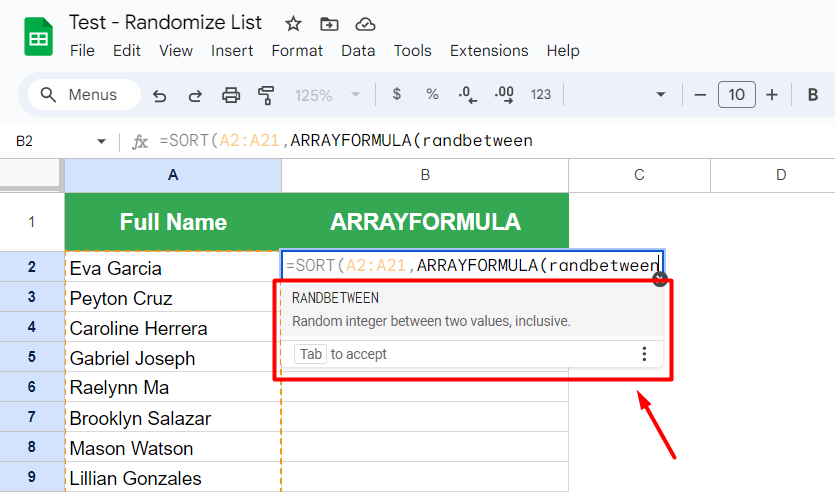• Type “sign
• Select the first option from the popup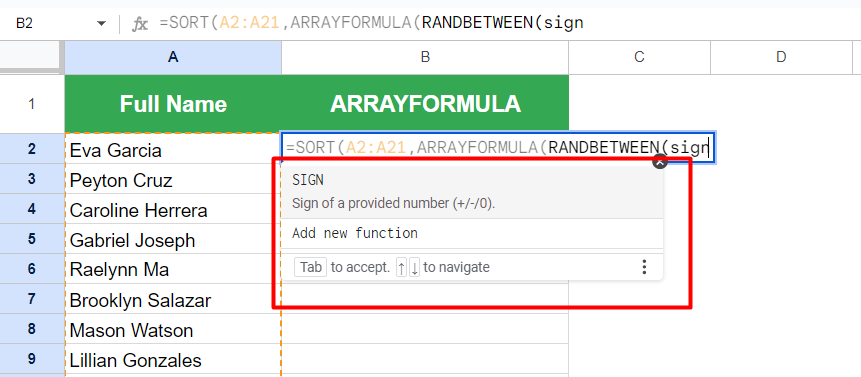• Type “row
• Select the first option from the popup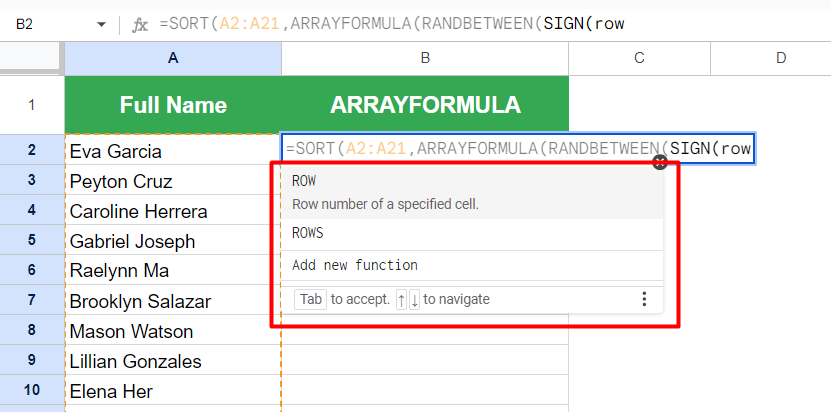• Provide the cell reference as “A2:A21” for the “cell_reference” argument of the ROW function
• Close the bracket for the ROW function using “)
• Close the bracket for the SIGN function using “)
• Now, you will be asked to provide the information for the second argument (“high”) of the RANDBETWEEN function. Let’s type “1000”
(Make sure to choose the number that will be greater than the size of the column range)
• Close the bracket for the RANDBETWEEN function using “)
• Close the bracket for the ARRAYFORMULA function using “)
• Next, you will be asked to replace the “is_ascending” argument with the proper information. Let us type “TRUE” and sort the cell range in ascending order
• Close the bracket for the SORT function using “)
• Press the “Enter” key

Here’s how our formula looks after following the above steps,

``=SORT(A2:A21,ARRAYFORMULA(RANDBETWEEN(SIGN(ROW(A2:A21)),1000)),TRUE)``

As you can see from the above GIF, after typing the formula, Google Sheets will instantly sort the names of the participants in a random order.

Note that this is a volatile formula due to the use of the RANDBETWEEN function. It means that if you perform any calculation, add entries, or perform any operation, the sorting will be automatically adjusted.

For example, let us add “asd” in the “D1“.

As seen in the above GIF, the sorting is automatically updated.

## Conclusion

The above methods use built-in functions and features by Google Sheets to randomize a list. Additionally, there are a few third-party add-ons that get the job done for you.

However, with the newly launched Randomize range feature, you don’t need anything else to randomize a list in Google Sheets.